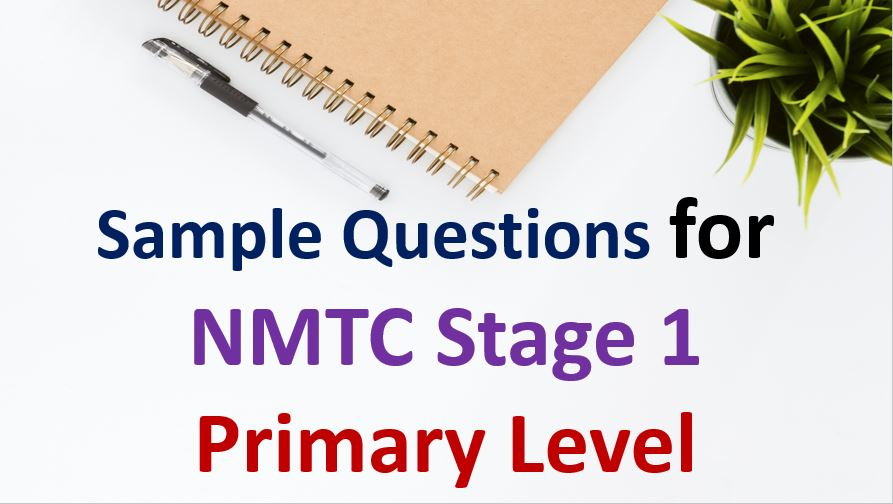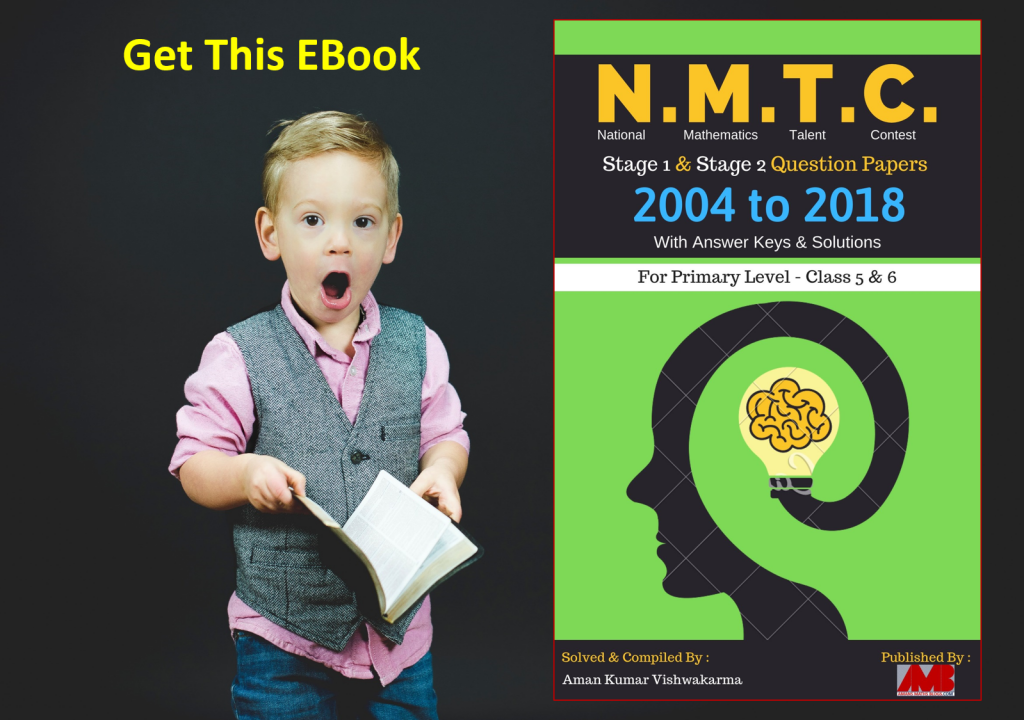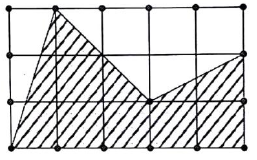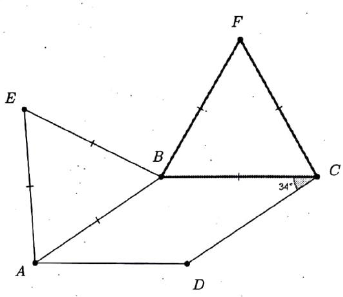Saturday, December 4, 2021
Home > NMTC > Sample Paper For NMTC Stage 1 Primary Level | Class 5 and 6

# Sample Paper For NMTC Stage 1 Primary Level | Class 5 and 6NMTC Stage 1 Sample Questions
Primary LevelSub Junior LevelJunior Level

Hi students, welcome to Amans Maths Blogs (AMB). On this post you will get Sample Paper For NMTC Stage 1 Primary Level (Class 5 and 6). The questions of this sample paper are published on this post, it will help you to understand the level of questions asked in NMTC Stage 1 in Primary Level. So lets start with the questions as below:Sample Paper For NMTC Stage 1 Class 5 and 6 Question No 1

Consider the numbers 2, 3, 4, 5. Form two digits numbers of different digits using these numbers. How many of them are odd?

Options

A. 4

B. 5

C. 6

D. 7

Sample Paper For NMTC Stage 1 Primary Level Question No 2

A is the sum of all even three digit numbers in which all the three digits are equal. B is the sum of all odd three digits numbers all the digits are equal. The value ofis

Options

A. 5/4

B. 4/3

C. 6/5

D. 7/6

Sample Paper For NMTC Stage 1 Class 5 and 6 Question No 3

21 rose plants, 42 sunflower plants and 56 dalia plants have to be planted in rows such that each row contains the same number of plants of one variety only. The minimum number of rows in which the above plants may be planted.

Options

A. 3

B. 15

C. 17

D. 21

Sample Paper For NMTC Stage 1 Primary Level Question No 4

The length and the breadth of a square are increased by 30% and 20% respectively. The area of the rectangle so formed exceeds the area of the square by

Options

A. 25%

B. 50%

C. 60%

D. 56%

Sample Paper For NMTC Stage 1 Class 5 and 6 Question No 5

ABCD is a rectangular play ground in which AB = 40 cm and BC = 30 m. The Physical director of the school gave punishment to two students Samrud and Saket.

Samrud has to start from A and go round the play ground along ABCDA twice.

Saket was asked to start from A, go along AM, MN, NC, CP, PQ and to A two times. MN is perpendicular to CD and PQ is perpendicular to DA. Then,Options

A. Samrud covers more distance than Saket

B. Saket covers more distance than Samrud

C. Samrud and Saket covers equal distance

D. Saket covers exactly twice the distance Samrud covers

Sample Paper For NMTC Stage 1 Class 5 and 6 Question No 6

B has Rs 5 more than C. A has Rs 14 more than B. Which of the following transaction makes equal money to all the three?

Options

A. A gives Rs 6 to B and B gives Rs 3 to C

B. A gives Rs 3 to B and C receives Rs 6 from A

C. A gives Rs 2 to C and B gives Rs 5 to C

D. A gives Rs 3 to B and C receives Rs 6 from A

Sample Paper For NMTC Stage 1 Primary Level Question No 7

A natural number ‘a’ is multiplied by 11 and 33 is added to it. It is divided by 9 and the remainder is zero. The smallest such natural number is

Options

A. 15

B. 3

C. 6

D. 1

Sample Paper For NMTC Stage 1 Class 5 and 6 Question No 8

n is a natural number such that (n + 1)(n + 2)(n + 3) = 201920192019. Then,

Options

A. n is two digit number

B. n is three digit number

C. No such n exist

D. n is a three digit number ending with 9

Sample Paper For NMTC Stage 1 Primary Level Question No 9

What fraction of the rectangle is shaded?Options

A. 1/2

B. 17/30

C. 5/8

D. 8/15

Sample Paper For NMTC Stage 1 Class 5 and 6 Question No 10

The first and the third digit of a five digit number d6d41 are the same. If the number is divisible by 9, the sum of its digit is

Options

A. 18

B. 36

C. 25

D. 27

Sample Paper For NMTC Stage 1 Primary Level Question No 11

The average of 10 consecutive odd numbers is 120. What is the average of the first five smallest numbers among them?

Options

A. 100

B. 105

C. 110

D. 115

Sample Paper For NMTC Stage 1 Class 5 and 6 Question No 12

ABC named high school has 1584 students 4/9 of the students are girls. The number of boys is N more than the number of girls in the school. The value of N is

Options

A. 704

B. 185

C. 176

D. 880

Sample Paper For NMTC Stage 1 Primary Level Question No 13

There is lengthy rope. Ram cuts one third of the rope. From the remaining Rahim cuts one fifth. The total length of the rope both cut is 861 meters. The length of the original uncut rope is

Options

A. 1845 m

B. 2015 m

C. 1256 m

D. 2235 m

Sample Paper For NMTC Stage 1 Class 5 and 6 Question No 14

Square papers of Black and White are arranged as shown. There are totally 80 squares. Find the number of white squares isOptions

A. 38

B. 39

C. 42

D. 43

Sample Paper For NMTC Stage 1 Primary Level Question No 15

A company promised Govind Rs. 21000 and a gift for working 2 years. But Govind left the job in 16 months and got Rs. 12500 and the gift as compensation. The gift of worth is Rs. _____.

Options

A. 1400

B. 1600

C. 1800

D. 1500

Sample Paper For NMTC Stage 1 Class 5 and 6 Question No 16

x and y are the digits of a two-digit number xy. x is greater than y by 3. When this two digit number is divided by the sum of its digits the quotient is 7 and the remainder is 3. The sum of the digits of the two digit number is ______.

Options

A. 6

B. 7

C. 9

D. 8

Sample Paper For NMTC Stage 1 Primary Level Question No 17

The number of perfect square number from 1 to 200 is

Options

A. 11

B. 14

C. 15

D. 16

Sample Paper For NMTC Stage 1 Class 5 and 6 Question No 18

Two ants start at A and walk at the same speed, one along the square and the other along the rectangle as shown below. The minimum distance (in cm) any one must cover before they meet again is _______.Options

A. 25 cm

B. 34 cm

C. 36 cm

D. 28 cm

Sample Paper For NMTC Stage 1 Primary Level Question No 19

If (1 + 1/2)(1 + 1/4)(1 + 1/6)(1 + 1/8)(1 + 1/10)(1 – 1/3)(1 – 1/5)(1 – 1/7)(1 – 1/9) = 1 + 1/n, then the value of n is

Options

A. 10

B. 11

C. 12

D. 13

Sample Paper For NMTC Stage 1 Class 5 and 6 Question No 20

In the figure below, ABCD is a rhombus. BFC and ABE are equilateral triangles. If ∠BCD = 34 degree, then ∠EFB isOptions

A. 43 degree

B. 54 degree

C. 63 degree

D. 73 degree

Sample Paper For NMTC Stage 1 Primary Level Question No 21

A sequence of numbers starts with 1, 2, 3 and every number of the sequence from the fourth term is the sum of the previous three numbers. The tenth number in the sequence is ______.

Options

A. 125

B. 230

C. 335

D. 423

Sample Paper For NMTC Stage 1 Class 5 and 6 Question No 22

Mahadevan conducted a problem solving session for a group of 18 primary class students. Seeing the graded performance, he distributed packets of biscuits to all the students. The total number of packets of biscuits distributed by Mahadevan is ______.

Options

A. 152

B. 287

C. 156

D. 252

Sample Paper For NMTC Stage 1 Primary Level Question No 23

Using the digits of the number 2016, two digits numbers of different digits are formed. The sum of all these numbers is

Options

A. 288

B. 287

C. 286

D. 285

1. C

2. A

3. A

4. D

5. C

6. D

7. C

8. C

9. B

10. D

11. D

12. C

13. A

14. B

15. D

16. B

17. B

18. C

19. A

20. A

21. B

22. D

23. A

NMTC Stage 1 Sample Questions